Tweeter Mids Woofer Subwoofer Passive Radiators no longer sold

# Accuton Thiel

Thiel & Partner knows to produce aluminiumoxyde cones.

What makes a Thiel ceramic loudspeaker driver so special? First and most important of all, it is the membrane itself. Only in a Thiel (ACCUTON) ceramic membrane you will find such desirable features as extreme cone ridigity and hardness which is needed for speed and accurate impulse response. The stiffness/weight ratio of this material is unsurpassed, the only exception being diamond. Also you will find a very high internal sound velocity which is important for a high breakup frequency point and extended linear range.

The unique electro-chemical manufacturing process out of aluminum foil leads to very thin, form-stable diaphragms of Al2O3 in Sapphire lattice with high internal damping, unusual for such hard material. This feature is very important and responsible for very low coloration and distortion, unachieved by other membrane materials. It allows the use of first order filters even in 2-way loudspeaker designs. The well balanced concave shape yields wide and uniform energy distribution, which is far more important than high on axis sound pressure. All mechanical parts are manufactured to high standards and very close tolerances, usually better than conventional drive units.

## Tweeteropen a bigger photo

## Accuton Thiel C25-6-012

order no. T-c25-6-012- not available
16,4 mm tweeter with ceramic cone. In linear operation the underhang voice coil remains completely within the magnetic field.
• power handling (continuous/programme) = 120 W
• frequency range = 2500-32000 Hz
• resonance frequency fs = 740 Hz
• impedance R = 8 Ohm
• sound pressure level SPL = 90 dB (2,83V; 1m)
• DC resistance Re = 6,2 Ohm
• force factor BL = 2,35 N/A
• voice coil inductance L = 0,02 mH
• effective piston radiating area Sd = 5,94 cm2
• effective mechanical mass incl. air load mms = 0,23 g
• total Q factor Qts = 0,38 (Qms=0,55, Qes=1,19)
• voice coil diameter = 16,4 mm
• maximum peak linear excursion vibration xlin = +/- 0,6 mm
• mounting diameter d = 64 mm
• overall diameter d = 82 mm
• mounting depth (not countersunk) t = 57 mm
• mass m = 0,58 kg
PDF data sheetopen a bigger photo

## Accuton Thiel C25-6-013

order no. T-c25-6-013- not available
16,4 mm tweeter with ceramic cone.
• power handling (continuous/programme) = 120 W
• frequency range = 2500-32000 Hz
• resonance frequency fs = 1450 Hz
• impedance R = 8 Ohm
• sound pressure level SPL = 92 dB (2,83V; 1m)
• DC resistance Re = 6,1 Ohm
• force factor BL = 2,47 N/A
• voice coil inductance L = 0,02 mH
• effective piston radiating area Sd = 5,94 cm2
• effective mechanical mass incl. air load mms = 0,18 g
• total Q factor Qts = 0,54 (Qms=0,80, Qes=1,63)
• voice coil diameter = 16,4 mm
• maximum peak linear excursion vibration xlin = +/- 0,15 mm
• mounting diameter d = 64 mm
• overall diameter d = 82 mm
• mounting depth (not countersunk) t = 67 mm
• mass m = 0,38 kg
PDF data sheet## Accuton Thiel C25-6-158

order no. T-c25-6-158       EUR 389,00excl. VAT: € 326.89 / \$ 359.58
28 mm tweeter with ceramic cone. The magnet system is based on Neodymium technology. In linear operation the underhang voice coil remains completely within the magnetic field.
• power handling (continuous/programme) = 100 W
• frequency range = 2500-30000 Hz
• resonance frequency fs = 810 Hz
• impedance R = 8 Ohm
• sound pressure level SPL = 92,5 dB (2,83V; 1m)
• DC resistance Re = 5,8 Ohm
• force factor BL = 3,27 N/A
• voice coil inductance L = 0,01 mH
• effective piston radiating area Sd = 5,94 cm2
• effective mechanical mass incl. air load mms = 0,17 g
• total Q factor Qts = 0,62 (Qms=2,68, Qes=0,81)
• voice coil diameter = 28 mm
• maximum peak linear excursion vibration xlin = +/- 1,2 mm
• mounting diameter d = 58 mm
• overall diameter d = 57,6 mm
• mounting depth (not countersunk) t = 28 mm
• mass m = 0,38 kg
PDF data sheetopen a bigger photo

## Accuton Thiel C30-6-024

order no. T-c30-6-024- not available
19,4 mm tweeter with ceramic cone. Voice coil windings immersed in magnetic fluid increase short term power handling capacity.
• power handling (continuous/programme) = 120 W
• frequency range = 1800-28000 Hz
• resonance frequency fs = 930 Hz
• impedance R = 8 Ohm
• sound pressure level SPL = 93 dB (2,83V; 1m)
• DC resistance Re = 6,0 Ohm
• force factor BL = 3,64 N/A
• voice coil inductance L = 0,03 mH
• effective piston radiating area Sd = 8,55 cm2
• effective mechanical mass incl. air load mms = 0,4 g
• total Q factor Qts = 0,69 (Qms=1,96, Qes=1,06)
• voice coil diameter = 19,4 mm
• maximum peak linear excursion vibration xlin = +/- 0,5 mm
• mounting diameter d = 64 mm
• overall diameter d = 82 mm
• mounting depth (not countersunk) t = 57 mm
• mass m = 0,44 kg
PDF data sheet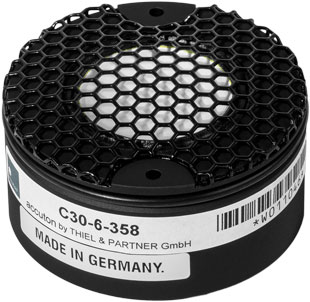## Accuton Thiel C30-6-358

order no. T-c30-6-358       EUR 428,00excl. VAT: € 359.66 / \$ 395.63
30 mm tweeter with ceramic cone. The magnet system is based on Neodymium technology. In linear operation the underhang voice coil remains completely within the magnetic field.
• power handling (continuous/programme) = 120 W
• frequency range = 2500-30000 Hz
• resonance frequency fs = 810 Hz
• impedance R = 8 Ohm
• sound pressure level SPL = 93,5 dB (2,83V; 1m)
• DC resistance Re = 5,8 Ohm
• force factor BL = 3,98 N/A
• voice coil inductance L = 0,01 mH
• effective piston radiating area Sd = 8,55 cm2
• effective mechanical mass incl. air load mms = 0,28 g
• total Q factor Qts = 0,61 (Qms=2,54, Qes=0,81)
• voice coil diameter = 30 mm
• maximum peak linear excursion vibration xlin = +/- 1,2 mm
• mounting diameter d = 58 mm
• overall diameter d = 57,6 mm
• mounting depth (not countersunk) t = 28 mm
• mass m = 0,38 kg
PDF data sheet

## Midsopen a bigger photo

## Accuton Thiel C90-6-078

order no. T-c90-6-078- not available
12 cm midrange driver with ceramic cone.
• power handling (continuous/programme) = 120 W
• frequency range = 200-5000 Hz
• resonance frequency fs = 100 Hz
• impedance R = 8 Ohm
• sound pressure level SPL = 88 dB (2,83V; 1m)
• DC resistance Re = 6,4 Ohm
• force factor BL = 4,3 N/A
• voice coil inductance L = 0,18 mH
• effective piston radiating area Sd = 86 cm2
• effective mechanical mass incl. air load mms = 6,96 g
• equivalent volume of compliance Vas = 3,9 l
• total Q factor Qts = 0,93 (Qms=2,4, Qes=1,52)
• voice coil diameter = 38 mm
• maximum peak linear excursion vibration xlin = +/- 3 mm
• mounting diameter d = 111 mm
• overall diameter d = 130 mm
• mounting depth (not countersunk) t = 64,5 mm
• mass m = 1,8 kg
PDF data sheetopen a bigger photo

recommended cabinet 1:
closed cabinet with 2,5 L volume
from 152/96 Hz (-3dB/-8dB)

recommended cabinet 2:
9,8 L volume bass reflex cabinet
with HP50 reflex tube, 5 cm long.
from 68/59 Hz (-3dB/-8dB).

## Accuton Thiel C90-6-079

order no. T-c90-6-079- not available
12 cm midrange driver with ceramic cone.
• power handling (continuous/programme) = 120 W
• frequency range = 250-5000 Hz
• resonance frequency fs = 105 Hz
• impedance R = 8 Ohm
• sound pressure level SPL = 87 dB (2,83V; 1m)
• DC resistance Re = 5,9 Ohm
• force factor BL = 3,84 N/A
• voice coil inductance L = 0,12 mH
• effective piston radiating area Sd = 75 cm2
• effective mechanical mass incl. air load mms = 5,7 g
• equivalent volume of compliance Vas = 3,2 l
• total Q factor Qts = 0,57 (Qms=0,9, Qes=1,52)
• voice coil diameter = 38 mm
• maximum peak linear excursion vibration xlin = +/- 2,6 mm
• mounting diameter d = 111 mm
• overall diameter d = 130 mm
• mounting depth (not countersunk) t = 64,5 mm
• mass m = 1,8 kg
PDF data sheetrecommended cabinet 1:
closed cabinet with 3,2 L volume
from 99/63 Hz (-3dB/-8dB)

recommended cabinet 2:
13,1 L volume bass reflex cabinet
with HP50 reflex tube, 12 cm long.
from 45/39 Hz (-3dB/-8dB).

## Accuton Thiel C90-6-724

order no. T-c90-6-724       EUR 519,00excl. VAT: € 436.13 / \$ 479.75
12 cm bass-midrange driver with ceramic cone. The magnet system is based on Neodymium technology. In linear operation the underhang voice coil remains completely within the magnetic field.
• power handling (continuous/programme) = 100 W
• frequency range = 70-5000 Hz
• resonance frequency fs = 65 Hz
• impedance R = 8 Ohm
• sound pressure level SPL = 89 dB (2,83V; 1m)
• DC resistance Re = 5,8 Ohm
• force factor BL = 6,1 N/A
• voice coil inductance L = 0,14 mH
• effective piston radiating area Sd = 81 cm2
• effective mechanical mass incl. air load mms = 8,9 g
• equivalent volume of compliance Vas = 5,1 l
• total Q factor Qts = 0,52 (Qms=5,47, Qes=0,58)
• voice coil diameter = 80 mm
• maximum peak linear excursion vibration xlin = +/- 4 mm
• mounting diameter d = 124 mm
• overall diameter d = 123,6 mm
• mounting depth (not countersunk) t = 55 mm
• mass m = 1,65 kg
PDF data sheetopen a bigger photo

recommended cabinet 1:
closed cabinet with 5,2 L volume
from 85/54 Hz (-3dB/-8dB)

recommended cabinet 2:
16 L volume bass reflex cabinet
with HP50 reflex tube, 15 cm long.
from 46/36 Hz (-3dB/-8dB).

## Accuton Thiel C158-8-085

order no. T-c158-8-085       EUR 309,00excl. VAT: € 259.66 / \$ 285.63
15 cm bass-midrange driver with ceramic cone.
• power handling (continuous/programme) = 100 W
• frequency range = 35-3000 Hz
• resonance frequency fs = 33 Hz
• impedance R = 8 Ohm
• sound pressure level SPL = 89 dB (2,83V; 1m)
• DC resistance Re = 7,8 Ohm
• force factor BL = 7,5 N/A
• voice coil inductance L = 0,54 mH
• effective piston radiating area Sd = 113 cm2
• effective mechanical mass incl. air load mms = 11,6 g
• equivalent volume of compliance Vas = 35 l
• total Q factor Qts = 0,31 (Qms=4,12, Qes=0,34)
• voice coil diameter = 25 mm
• maximum peak linear excursion vibration xlin = +/- 5 mm
• mounting diameter d = 137 mm
• overall diameter d = 158 mm
• mounting depth (not countersunk) t = 82 mm
• mass m = 1,65 kg
PDF data sheet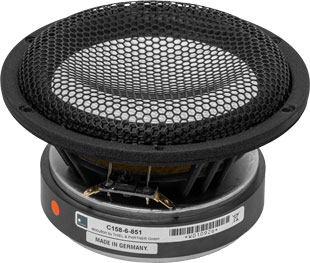open a bigger photo

recommended cabinet 1:
closed cabinet with 3,1 L volume
from 85/54 Hz (-3dB/-8dB)

recommended cabinet 2:
8,6 L volume bass reflex cabinet
with HP50 reflex tube, 31 cm long.
from 48/37 Hz (-3dB/-8dB).

## Accuton Thiel C158-6-851

order no. T-c158-6-851       EUR 328,00excl. VAT: € 275.63 / \$ 303.19
15 cm bass-midrange driver with ceramic cone.
• power handling (continuous/programme) = 120 W
• frequency range = 30-3000 Hz
• resonance frequency fs = 30 Hz
• impedance R = 8 Ohm
• sound pressure level SPL = 88 dB (2,83V; 1m)
• DC resistance Re = 6,3 Ohm
• force factor BL = 8,7 N/A
• voice coil inductance L = 0,73 mH
• effective piston radiating area Sd = 113 cm2
• effective mechanical mass incl. air load mms = 19 g
• equivalent volume of compliance Vas = 26,4 l
• total Q factor Qts = 0,28 (Qms=5,1, Qes=0,3)
• voice coil diameter = 38 mm
• maximum peak linear excursion vibration xlin = +/- 5 mm
• mounting diameter d = 137 mm
• overall diameter d = 158 mm
• mounting depth (not countersunk) t = 84 mm
• mass m = 2,28 kg
PDF data sheet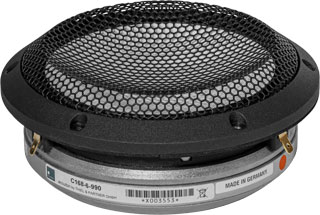recommended cabinet 1:
closed cabinet with 0,84 L volume
from 206/130 Hz (-3dB/-8dB)

recommended cabinet 2:
1,7 L volume bass reflex cabinet
with HP50 reflex tube, 27 cm long.
from 132/98 Hz (-3dB/-8dB).

## Accuton Thiel C168-6-990

order no. T-c168-6-990       EUR 878,00excl. VAT: € 737.82 / \$ 811.60
16 cm midrange driver with ceramic cone. The magnet system is based on Neodymium technology. In linear operation the underhang voice coil remains completely within the magnetic field.
• power handling (continuous/programme) = 120 W
• frequency range = 200-6000 Hz
• resonance frequency fs = 55 Hz
• impedance R = 8 Ohm
• sound pressure level SPL = 93 dB (2,83V; 1m)
• DC resistance Re = 5,4 Ohm
• force factor BL = 11,66 N/A
• voice coil inductance L = 0,06 mH
• effective piston radiating area Sd = 133 cm2
• effective mechanical mass incl. air load mms = 16,1 g
• equivalent volume of compliance Vas = 13,1 l
• total Q factor Qts = 0,21 (Qms=4,76, Qes=0,22)
• maximum peak linear excursion vibration xlin = +/- 3 mm
• mounting diameter d = 147 mm
• overall diameter d = 168 mm
• mounting depth (not countersunk) t = 49,5 mm
• mass m = 1,9 kg
PDF data sheetopen a bigger photo

recommended cabinet 1:
closed cabinet with 1,3 L volume
from 188/119 Hz (-3dB/-8dB)

recommended cabinet 2:
3,1 L volume bass reflex cabinet
with HP50 reflex tube, 16 cm long.
from 114/85 Hz (-3dB/-8dB).

## Accuton Thiel C173-6-090

order no. T-c173-6-090- not available
16 cm midrange driver with ceramic cone.
• power handling (continuous/programme) = 120 W
• frequency range = 80-3200 Hz
• resonance frequency fs = 57 Hz
• impedance R = 8 Ohm
• sound pressure level SPL = 93 dB (2,83V; 1m)
• DC resistance Re = 6,61 Ohm
• force factor BL = 11,6 N/A
• voice coil inductance L = 0,19 mH
• effective piston radiating area Sd = 133 cm2
• effective mechanical mass incl. air load mms = 14 g
• equivalent volume of compliance Vas = 15,5 l
• total Q factor Qts = 0,24 (Qms=3,75, Qes=0,26)
• voice coil diameter = 55 mm
• maximum peak linear excursion vibration xlin = +/- 5 mm
• mounting diameter d = 145,6 mm
• overall diameter d = 173 mm
• mounting depth (not countersunk) t = 96,35 mm
• mass m = 3,44 kg
PDF data sheetopen a bigger photo

recommended cabinet 1:
closed cabinet with 1,2 L volume
from 179/113 Hz (-3dB/-8dB)

recommended cabinet 2:
1,9 L volume bass reflex cabinet
with HP50 reflex tube, 34 cm long.
from 126/93 Hz (-3dB/-8dB).

## Accuton Thiel C173-6-096

order no. T-c173-6-096       EUR 819,00excl. VAT: € 688.24 / \$ 757.06
16 cm bass-midrange driver with ceramic cone.
• power handling (continuous/programme) = 120 W
• frequency range = 40-3000 Hz
• resonance frequency fs = 39 Hz
• impedance R = 8 Ohm
• sound pressure level SPL = 94 dB (2,83V; 1m)
• DC resistance Re = 6,61 Ohm
• force factor BL = 11,5 N/A
• voice coil inductance L = 0,25 mH
• effective piston radiating area Sd = 130 cm2
• effective mechanical mass incl. air load mms = 14,5 g
• equivalent volume of compliance Vas = 28 l
• total Q factor Qts = 0,17 (Qms=3,9, Qes=0,18)
• voice coil diameter = 55 mm
• maximum peak linear excursion vibration xlin = +/- 5 mm
• mounting diameter d = 145,60 mm
• overall diameter d = 173 mm
• mounting depth (not countersunk) t = 96,35 mm
• mass m = 3,44 kg
PDF data sheetopen a bigger photo

recommended cabinet 1:
closed cabinet with 1,2 L volume
from 165/104 Hz (-3dB/-8dB)

recommended cabinet 2:
2 L volume bass reflex cabinet
with HP50 reflex tube, 38 cm long.
from 117/86 Hz (-3dB/-8dB).

## Accuton Thiel C173-6-096e

order no. T-c173-6-096e- not available
16 cm bass-midrange driver with ceramic cone.
• power handling (continuous/programme) = 120 W
• frequency range = 40-3000 Hz
• resonance frequency fs = 36 Hz
• impedance R = 8 Ohm
• sound pressure level SPL = 92,5 dB (2,83V; 1m)
• DC resistance Re = 6,61 Ohm
• force factor BL = 11,5 N/A
• voice coil inductance L = 0,20 mH
• effective piston radiating area Sd = 130 cm2
• effective mechanical mass incl. air load mms = 15,5 g
• equivalent volume of compliance Vas = 29,8 l
• total Q factor Qts = 0,17 (Qms=4,07, Qes=0,18)
• voice coil diameter = 55 mm
• maximum peak linear excursion vibration xlin = +/- 5 mm
• mounting diameter d = 145,60 mm
• overall diameter d = 173 mm
• mounting depth (not countersunk) t = 96,35 mm
• mass m = 3,44 kg
PDF data sheet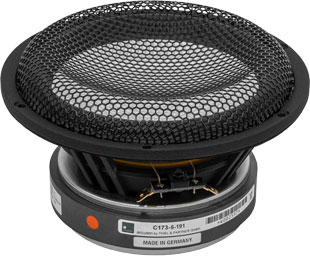open a bigger photo

recommended cabinet 1:
closed cabinet with 4,9 L volume
from 85/54 Hz (-3dB/-8dB)

recommended cabinet 2:
14,8 L volume bass reflex cabinet
with HP50 reflex tube, 16 cm long.
from 46/36 Hz (-3dB/-8dB).

## Accuton Thiel C173-6-191

order no. T-c173-6-191       EUR 339,00excl. VAT: € 284.87 / \$ 313.36
16 cm bass-midrange driver with ceramic cone.
• power handling (continuous/programme) = 120 W
• frequency range = 33-2500 Hz
• resonance frequency fs = 33 Hz
• impedance R = 8 Ohm
• sound pressure level SPL = 88 dB (2,83V; 1m)
• DC resistance Re = 5,8 Ohm
• force factor BL = 8,5 N/A
• voice coil inductance L = 0,7 mH
• effective piston radiating area Sd = 130 cm2
• effective mechanical mass incl. air load mms = 18 g
• equivalent volume of compliance Vas = 33 l
• total Q factor Qts = 0,31 (Qms=5,3, Qes=0,33)
• voice coil diameter = 38 mm
• maximum peak linear excursion vibration xlin = +/- 5 mm
• mounting diameter d = 145,6 mm
• overall diameter d = 173 mm
• mounting depth (not countersunk) t = 94,5 mm
• mass m = 2,28 kg
PDF data sheetopen a bigger photo

recommended cabinet 1:
closed cabinet with 5,3 L volume
from 82/52 Hz (-3dB/-8dB)

recommended cabinet 2:
16 L volume bass reflex cabinet
with HP50 reflex tube, 15 cm long.
from 44/34 Hz (-3dB/-8dB).

## Accuton Thiel C173-6-191e

order no. T-c173-6-191e- not available
16 cm bass-midrange driver with ceramic cone.
• power handling (continuous/programme) = 120 W
• frequency range = 33-2500 Hz
• resonance frequency fs = 33 Hz
• impedance R = 8 Ohm
• sound pressure level SPL = 88 dB (2,83V; 1m)
• DC resistance Re = 5,8 Ohm
• force factor BL = 8,5 N/A
• voice coil inductance L = 0,7 mH
• effective piston radiating area Sd = 132 cm2
• effective mechanical mass incl. air load mms = 19,2 g
• equivalent volume of compliance Vas = 33 l
• total Q factor Qts = 0,32 (Qms=5,1, Qes=0,34)
• voice coil diameter = 38 mm
• maximum peak linear excursion vibration xlin = +/- 5 mm
• mounting diameter d = 145,6 mm
• overall diameter d = 173 mm
• mounting depth (not countersunk) t = 94,5 mm
• mass m = 2,28 kg
PDF data sheetopen a bigger photo

recommended cabinet 1:
closed cabinet with 4,5 L volume
from 85/54 Hz (-3dB/-8dB)

recommended cabinet 2:
13,4 L volume bass reflex cabinet
with HP50 reflex tube, 18 cm long.
from 47/36 Hz (-3dB/-8dB).

## Accuton Thiel C173-11-191e

order no. T-c173-11-191e       EUR 429,00excl. VAT: € 360.50 / \$ 396.55
16 cm bass-midrange driver with ceramic cone.
• power handling (continuous/programme) = 120 W
• frequency range = 33-2500 Hz
• resonance frequency fs = 32 Hz
• impedance R = 12 Ohm
• sound pressure level SPL = 86 dB (2,83V; 1m)
• DC resistance Re = 10,9 Ohm
• force factor BL = 11,1 N/A
• voice coil inductance L = 1,1 mH
• effective piston radiating area Sd = 132 cm2
• effective mechanical mass incl. air load mms = 17 g
• equivalent volume of compliance Vas = 33 l
• total Q factor Qts = 0,3 (Qms=5, Qes=0,32)
• voice coil diameter = 38 mm
• maximum peak linear excursion vibration xlin = +/- 5 mm
• mounting diameter d = 145,6 mm
• overall diameter d = 173 mm
• mounting depth (not countersunk) t = 94,5 mm
• mass m = 2,28 kg

## Woofer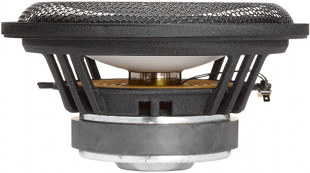open a bigger photo

recommended cabinet 1:
closed cabinet with 18 L volume
from 61/38 Hz (-3dB/-8dB)

recommended cabinet 2:
63 L volume bass reflex cabinet
with HP100 reflex tube, 31 cm long.
from 31/25 Hz (-3dB/-8dB).

## Accuton Thiel C220-6-221

order no. T-c220-6-221       EUR 489,00excl. VAT: € 410.92 / \$ 452.02
21 cm bass driver with ceramic cone.
• power handling (continuous/programme) = 150 W
• frequency range = 32-2000 Hz
• resonance frequency fs = 28 Hz
• impedance R = 8 Ohm
• sound pressure level SPL = 90 dB (2,83V; 1m)
• DC resistance Re = 5,8 Ohm
• force factor BL = 8,9 N/A
• voice coil inductance L = 0,8 mH
• effective piston radiating area Sd = 227 cm2
• effective mechanical mass incl. air load mms = 30,9 g
• equivalent volume of compliance Vas = 79 l
• total Q factor Qts = 0,37 (Qms=7,4, Qes=0,39)
• voice coil diameter = 38 mm
• maximum peak linear excursion vibration xlin = +/- 5 mm
• mounting diameter d = 190,5 mm
• overall diameter d = 220 mm
• mounting depth (not countersunk) t = 105 mm
• mass m = 2,10 kg
PDF data sheet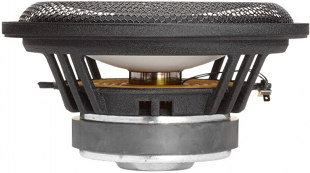open a bigger photo

recommended cabinet 1:
closed cabinet with 26 L volume
from 51/32 Hz (-3dB/-8dB)

recommended cabinet 2:
98 L volume bass reflex cabinet
with HP100 reflex tube, 28 cm long.
from 25/20 Hz (-3dB/-8dB).

## Accuton Thiel C220-11-221

order no. T-c220-11-221       EUR 509,00excl. VAT: € 427.73 / \$ 470.50
21 cm bass driver with ceramic cone.
• power handling (continuous/programme) = 150 W
• frequency range = 32-2000 Hz
• resonance frequency fs = 25,2 Hz
• impedance R = 12 Ohm
• sound pressure level SPL = 88 dB (2,83V; 1m)
• DC resistance Re = 10,9 Ohm
• force factor BL = 11,1 N/A
• voice coil inductance L = 1,2 mH
• effective piston radiating area Sd = 227 cm2
• effective mechanical mass incl. air load mms = 30,6 g
• equivalent volume of compliance Vas = 95 l
• total Q factor Qts = 0,4 (Qms=6,74, Qes=0,43)
• voice coil diameter = 38 mm
• maximum peak linear excursion vibration xlin = +/- 5 mm
• mounting diameter d = 190,5 mm
• overall diameter d = 220 mm
• mounting depth (not countersunk) t = 105 mm
• mass m = 2,10 kg
PDF data sheetopen a bigger photo

recommended cabinet 1:
closed cabinet with 6 L volume
from 99/62 Hz (-3dB/-8dB)

recommended cabinet 2:
10,8 L volume bass reflex cabinet
with HP70 reflex tube, 35 cm long.
from 66/49 Hz (-3dB/-8dB).

## Accuton Thiel C220-6-222

order no. T-c220-6-222       EUR 999,00excl. VAT: € 839.50 / \$ 923.45
21 cm bass driver with ceramic cone.
• power handling (continuous/programme) = 150 W
• frequency range = 32-2500 Hz
• resonance frequency fs = 24 Hz
• impedance R = 8 Ohm
• sound pressure level SPL = 93 dB (2,83V; 1m)
• DC resistance Re = 6,7 Ohm
• force factor BL = 11,5 N/A
• voice coil inductance L = 0,42 mH
• effective piston radiating area Sd = 224 cm2
• effective mechanical mass incl. air load mms = 27 g
• equivalent volume of compliance Vas = 115 l
• total Q factor Qts = 0,19 (Qms=4,4, Qes=0,20)
• voice coil diameter = 55 mm
• maximum peak linear excursion vibration xlin = +/- 5 mm
• mounting diameter d = 190,5 mm
• overall diameter d = 220 mm
• mounting depth (not countersunk) t = 107 mm
• mass m = 3,8 kg
PDF data sheetopen a bigger photo

recommended cabinet 1:
closed cabinet with 24 L volume
from 47/30 Hz (-3dB/-8dB)

recommended cabinet 2:
85 L volume bass reflex cabinet
with HP100 reflex tube, 41 cm long.
from 24/19 Hz (-3dB/-8dB).

## Accuton Thiel S220-6-221

order no. T-s220-6-221       EUR 477,00excl. VAT: € 400.84 / \$ 440.92
21 cm bass driver with sandwich cone.
• power handling (continuous/programme) = 150 W
• frequency range = 31-1000 Hz
• resonance frequency fs = 21 Hz
• impedance R = 8 Ohm
• sound pressure level SPL = 89 dB (2,83V; 1m)
• DC resistance Re = 5,8 Ohm
• force factor BL = 8,9 N/A
• voice coil inductance L = 0,8 mH
• effective piston radiating area Sd = 227 cm2
• effective mechanical mass incl. air load mms = 33,6 g
• equivalent volume of compliance Vas = 116 l
• total Q factor Qts = 0,36 (Qms=6,6, Qes=0,38)
• voice coil diameter = 38 mm
• maximum peak linear excursion vibration xlin = +/- 5 mm
• mounting diameter d = 190,5 mm
• overall diameter d = 220 mm
• mounting depth (not countersunk) t = 105 mm
• mass m = 2,10 kg
PDF data sheet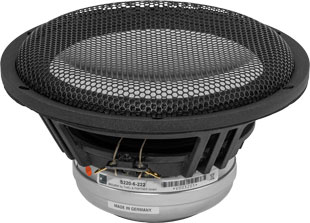open a bigger photo

recommended cabinet 1:
closed cabinet with 8,1 L volume
from 82/52 Hz (-3dB/-8dB)

recommended cabinet 2:
15 L volume bass reflex cabinet
with HP70 reflex tube, 36 cm long.
from 54/40 Hz (-3dB/-8dB).

## Accuton Thiel S220-6-222

order no. T-s220-6-222       EUR 979,00excl. VAT: € 822.69 / \$ 904.96
21 cm bass driver with sandwich cone.
• power handling (continuous/programme) = 150 W
• frequency range = 31-1000 Hz
• resonance frequency fs = 21 Hz
• impedance R = 8 Ohm
• sound pressure level SPL = 92,5 dB (2,83V; 1m)
• DC resistance Re = 6,7 Ohm
• force factor BL = 11,5 N/A
• voice coil inductance L = 0,42 mH
• effective piston radiating area Sd = 224 cm2
• effective mechanical mass incl. air load mms = 30 g
• equivalent volume of compliance Vas = 139 l
• total Q factor Qts = 0,2 (Qms=4,4, Qes=0,21)
• voice coil diameter = 55 mm
• maximum peak linear excursion vibration xlin = +/- 5 mm
• mounting diameter d = 190,5 mm
• overall diameter d = 220 mm
• mounting depth (not countersunk) t = 107 mm
• mass m = 3,8 kg
PDF data sheet

## Subwoofer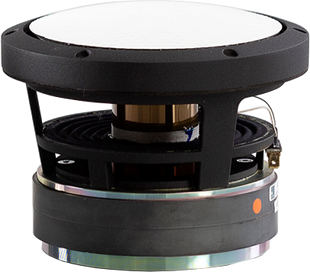recommended cabinet 1:
closed cabinet with 7 L volume
from 62/39 Hz (-3dB/-8dB)

recommended cabinet 2:
24 L volume bass reflex cabinet
with HP50 reflex tube, 20 cm long.
from 32/25 Hz (-3dB/-8dB).

## Accuton Thiel AS168-9-470

order no. T-as168-9-470       EUR 579,00excl. VAT: € 486.55 / \$ 535.21
16 cm bass driver with sandwich cone.
• power handling (continuous/programme) = 250 W
• frequency range = 35-1000 Hz
• resonance frequency fs = 27 Hz
• impedance R = 12 Ohm
• sound pressure level SPL = 84 dB (2,83V; 1m)
• DC resistance Re = 9 Ohm
• force factor BL = 12,1 N/A
• voice coil inductance L = 1 mH
• effective piston radiating area Sd = 161 cm2
• effective mechanical mass incl. air load mms = 36 g
• equivalent volume of compliance Vas = 35,5 l
• total Q factor Qts = 0,35 (Qms=4,35, Qes=0,38)
• voice coil diameter = 47 mm
• maximum peak linear excursion vibration xlin = +/- 8 mm
• mounting diameter d = 148 mm
• overall diameter d = 168 mm
• mounting depth (not countersunk) t = 118 mm
• mass m = 4,2 kg
PDF data sheetrecommended cabinet 1:
closed cabinet with 9,5 L volume
from 61/39 Hz (-3dB/-8dB)

recommended cabinet 2:
37 L volume bass reflex cabinet
with HP70 reflex tube, 24 cm long.
from 29/24 Hz (-3dB/-8dB).

## Accuton Thiel AS190-9-251

order no. T-as190-9-251       EUR 979,00excl. VAT: € 822.69 / \$ 904.96
18 cm bass driver with sandwich cone.
• power handling (continuous/programme) = 250 W
• frequency range = 30-1000 Hz
• resonance frequency fs = 33,4 Hz
• impedance R = 12 Ohm
• sound pressure level SPL = 84 dB (2,83V; 1m)
• DC resistance Re = 8,6 Ohm
• force factor BL = 13,5 N/A
• voice coil inductance L = 1,05 mH
• effective piston radiating area Sd = 202 cm2
• effective mechanical mass incl. air load mms = 49,4 g
• equivalent volume of compliance Vas = 26,7 l
• total Q factor Qts = 0,44 (Qms=4,75, Qes=0,49)
• voice coil diameter = 78 mm
• maximum peak linear excursion vibration xlin = +/- 9 mm
• mounting diameter d = 170 mm
• overall diameter d = 190 mm
• mounting depth (not countersunk) t = 137 mm
• mass m = 7 kg
PDF data sheetrecommended cabinet 1:
closed cabinet with 25 L volume
from 49/31 Hz (-3dB/-8dB)

recommended cabinet 2:
87 L volume bass reflex cabinet
with HP100 reflex tube, 36 cm long.
from 25/20 Hz (-3dB/-8dB).

## Accuton Thiel AS250-8-552

order no. T-as250-8-552       EUR 1429,00excl. VAT: € 1200.84 / \$ 1320.92
24 cm bass driver with sandwich cone.
• power handling (continuous/programme) = 300 W
• frequency range = 20-600 Hz
• resonance frequency fs = 22 Hz
• impedance R = 8 Ohm
• sound pressure level SPL = 87 dB (2,83V; 1m)
• DC resistance Re = 7,3 Ohm
• force factor BL = 15,1 N/A
• voice coil inductance L = 0,67 mH
• effective piston radiating area Sd = 382 cm2
• effective mechanical mass incl. air load mms = 92 g
• equivalent volume of compliance Vas = 119 l
• total Q factor Qts = 0,35 (Qms=5, Qes=0,38)
• voice coil diameter = 114 mm
• maximum peak linear excursion vibration xlin = +/- 9 mm
• mounting diameter d = 230 mm
• overall diameter d = 250 mm
• mounting depth (not countersunk) t = 141 mm
• mass m = 11,5 kg
PDF data sheetopen a bigger photo

recommended cabinet 1:
closed cabinet with 23 L volume
from 61/39 Hz (-3dB/-8dB)

recommended cabinet 2:
58 L volume bass reflex cabinet
with HP100 reflex tube, 35 cm long.
from 36/27 Hz (-3dB/-8dB).

## Accuton Thiel S280-6-282

order no. T-s280-6-282       EUR 1379,00excl. VAT: € 1158.82 / \$ 1274.71
27 cm bass driver with sandwich cone.
• power handling (continuous/programme) = 150 W
• frequency range = 20-600 Hz
• resonance frequency fs = 20 Hz
• impedance R = 8 Ohm
• sound pressure level SPL = 93 dB (2,83V; 1m)
• DC resistance Re = 6,6 Ohm
• force factor BL = 14,1 N/A
• voice coil inductance L = 0,64 mH
• effective piston radiating area Sd = 408 cm2
• effective mechanical mass incl. air load mms = 65 g
• equivalent volume of compliance Vas = 226 l
• total Q factor Qts = 0,26 (Qms=4,43, Qes=0,28)
• voice coil diameter = 55 mm
• maximum peak linear excursion vibration xlin = +/- 6 mm
• mounting diameter d = 251,2 mm
• overall diameter d = 280 mm
• mounting depth (not countersunk) t = 131,95 mm
• mass m = 4,80 kg
PDF data sheetopen a bigger photo

recommended cabinet 1:
closed cabinet with 21 L volume
from 63/40 Hz (-3dB/-8dB)

recommended cabinet 2:
51 L volume bass reflex cabinet
with HP70 reflex tube, 16 cm long.
from 38/28 Hz (-3dB/-8dB).

## Accuton Thiel S280-18-282

order no. T-s280-18-282       EUR 1389,00excl. VAT: € 1167.23 / \$ 1283.95
27 cm bass driver with sandwich cone.
• power handling (continuous/programme) = 150 W
• frequency range = 20-600 Hz
• resonance frequency fs = 20 Hz
• impedance R = 20 Ohm
• sound pressure level SPL = 88,5 dB (2,83V; 1m)
• DC resistance Re = 18,5 Ohm
• force factor BL = 23,7 N/A
• voice coil inductance L = 2,25 mH
• effective piston radiating area Sd = 408 cm2
• effective mechanical mass incl. air load mms = 63 g
• equivalent volume of compliance Vas = 226 l
• total Q factor Qts = 0,26 (Qms=6,74, Qes=0,27)
• voice coil diameter = 55 mm
• maximum peak linear excursion vibration xlin = +/- 6 mm
• mounting diameter d = 251,2 mm
• overall diameter d = 280 mm
• mounting depth (not countersunk) t = 131,95 mm
• mass m = 3,8 kg
PDF data sheetopen a bigger photo

## Accuton Thiel P173

order no. T-p173       EUR 109,00excl. VAT: € 91.60 / \$ 100.76
• resonance frequency fs = 41 Hz
• effective piston radiating area Sd = 130 cm2
• effective mechanical mass incl. air load mms = 15 g
• equivalent volume of compliance Vas = 24 l
• mounting diameter d = 145,6 mm
• overall diameter d = 173 mm
• mounting depth (not countersunk) t = 62 mm
PDF data sheet## Accuton Thiel ASP190

order no. T-asp190       EUR 659,00excl. VAT: € 553.78 / \$ 609.16
• resonance frequency fs = 33 Hz
• effective piston radiating area Sd = 202 cm2
• effective mechanical mass incl. air load mms = 50 g
• equivalent volume of compliance Vas = 27 l
• mounting diameter d = 170 mm
• overall diameter d = 190 mm
• mounting depth (not countersunk) t = 85 mm
PDF data sheetopen a bigger photo

## Accuton Thiel P220

order no. T-p220       EUR 129,00excl. VAT: € 108.40 / \$ 119.24
• resonance frequency fs = 41 Hz
• effective piston radiating area Sd = 224 cm2
• effective mechanical mass incl. air load mms = 23,4 g
• equivalent volume of compliance Vas = 26,5 l
• mounting diameter d = 190,5 mm
• overall diameter d = 220 mm
• mounting depth (not countersunk) t = 76 mm
PDF data sheet## Accuton Thiel ASP250

order no. T-asp250       EUR 699,00excl. VAT: € 587.39 / \$ 646.13
• resonance frequency fs = 22 Hz
• effective piston radiating area Sd = 382 cm2
• effective mechanical mass incl. air load mms = 93 g
• equivalent volume of compliance Vas = 119 l
• mounting diameter d = 230 mm
• overall diameter d = 250 mm
• mounting depth (not countersunk) t = 85 mm
PDF data sheet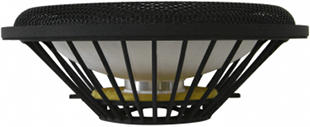open a bigger photo

## Accuton Thiel P280

order no. T-p280       EUR 349,00excl. VAT: € 293.28 / \$ 322.61
• resonance frequency fs = 21 Hz
• effective piston radiating area Sd = 408 cm2
• effective mechanical mass incl. air load mms = 63 g
• equivalent volume of compliance Vas = 226 l
• mounting diameter d = 251,2 mm
• overall diameter d = 280 mm
• mounting depth (not countersunk) t = 131,95 mm
PDF data sheet

## no longer soldopen a bigger photo

## Accuton Thiel C50-8-044 (C² 44) Ohne Koppelvolumen für Bausatz Chorda

order no. T-c448oV       EUR 288,00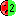excl. VAT: € 242.02 / \$ 266.22
30,5 mm tweeter with ceramic cone. Voice coil windings immersed in magnetic fluid increase short term power handling capacity.
• power handling (continuous/programme) = 120 W
• frequency range = 300-23000 Hz
• resonance frequency fs = 260 Hz
• impedance R = 8 Ohm
• sound pressure level SPL = 86 dB (2,83V; 1m)
• DC resistance Re = 7,7 Ohm
• effective piston radiating area Sd = 23,8 cm2
• total Q factor Qts = 0,62 (Qms=0,95, Qes=1,8)
• voice coil diameter = 30,5 mm
• mounting diameter d = 88 mm
• overall diameter d = 106 mm
• mounting depth (not countersunk) t = 33 mm
• mass m = 0,7 kgopen a bigger photo

## Accuton Thiel C50-8-044 (C² 44)

order no. T-c50-8-044       EUR 288,00excl. VAT: € 242.02 / \$ 266.22
30,5 mm tweeter with ceramic cone. Voice coil windings immersed in magnetic fluid increase short term power handling capacity.
• power handling (continuous/programme) = 120 W
• frequency range = 800-12000 Hz
• resonance frequency fs = 380 Hz
• impedance R = 8 Ohm
• sound pressure level SPL = 89 dB (2,83V; 1m)
• DC resistance Re = 8,0 Ohm
• force factor BL = 3,13 N/A
• voice coil inductance L = 0,04 mH
• effective piston radiating area Sd = 23,8 cm2
• effective mechanical mass incl. air load mms = 1,19 g
• total Q factor Qts = 0,74 (Qms=1,08, Qes=2,32)
• voice coil diameter = 30,5 mm
• maximum peak linear excursion vibration xlin = +/- 0,75 mm
• mounting diameter d = 88 mm
• overall diameter d = 106 mm
• mounting depth (not countersunk) t = 67,5 mm
• mass m = 0,88 kg
PDF data sheetopen a bigger photo

recommended cabinet 1:
closed cabinet with 3,6 L volume
from 97/61 Hz (-3dB/-8dB)

recommended cabinet 2:
9,5 L volume bass reflex cabinet
with HP50 reflex tube, 20 cm long.
from 56/42 Hz (-3dB/-8dB).

## Accuton Thiel C173-T6-95 (C² 95/T6)

order no. T-c295t6- not available
16 cm bass-midrange driver with ceramic cone.
• power handling (continuous/programme) = 120 W
• frequency range = 35-2500 Hz
• resonance frequency fs = 33 Hz
• impedance R = 8 Ohm
• sound pressure level SPL = 88 dB (2,83V; 1m)
• DC resistance Re = 5,8 Ohm
• force factor BL = 8,5 N/A
• voice coil inductance L = 0,7 mH
• effective piston radiating area Sd = 130 cm2
• effective mechanical mass incl. air load mms = 17 g
• equivalent volume of compliance Vas = 33 l
• total Q factor Qts = 0,27 (Qms=4,9, Qes=0,29)
• voice coil diameter = 38 mm
• maximum peak linear excursion vibration xlin = +/- 5 mm
• mounting diameter d = 145,60 mm
• overall diameter d = 173 mm
• mounting depth (not countersunk) t = 94,50 mm
PDF data sheetopen a bigger photo

recommended cabinet 1:
closed cabinet with 1,2 L volume
from 179/113 Hz (-3dB/-8dB)

recommended cabinet 2:
1,9 L volume bass reflex cabinet
with HP50 reflex tube, 34 cm long.
from 126/93 Hz (-3dB/-8dB).

## Accuton Thiel C173N-T6-096

order no. T-c173nt6       EUR 427,00excl. VAT: € 358.82 / \$ 394.71
16 cm bass-midrange driver with ceramic cone.
• power handling (continuous/programme) = 120 W
• frequency range = 40-3000 Hz
• resonance frequency fs = 39 Hz
• impedance R = 8 Ohm
• sound pressure level SPL = 94 dB (2,83V; 1m)
• DC resistance Re = 6,61 Ohm
• force factor BL = 11,5 N/A
• voice coil inductance L = 0,25 mH
• effective piston radiating area Sd = 130 cm2
• effective mechanical mass incl. air load mms = 14,5 g
• equivalent volume of compliance Vas = 28 l
• total Q factor Qts = 0,17 (Qms=3,9, Qes=0,18)
• voice coil diameter = 55 mm
• maximum peak linear excursion vibration xlin = +/- 5 mm
• mounting diameter d = 145,60 mm
• overall diameter d = 173 mm
• mounting depth (not countersunk) t = 96,35 mm
• mass m = 3,44 kgrecommended cabinet 1:
closed cabinet with 17 L volume
from 56/35 Hz (-3dB/-8dB)

recommended cabinet 2:
66 L volume bass reflex cabinet
with HP70 reflex tube, 15 cm long.
from 27/22 Hz (-3dB/-8dB).

## Accuton Thiel C173-6-92 (C² 92/6)

order no. T-c2926       EUR 217,00excl. VAT: € 182.35 / \$ 200.59
16 cm bass-midrange driver with ceramic cone.
• power handling (continuous/programme) = 130 W
• frequency range = 30-3000 Hz
• resonance frequency fs = 29 Hz
• impedance R = 8 Ohm
• sound pressure level SPL = 86,1 dB (2,83V; 1m)
• DC resistance Re = 6,1 Ohm
• force factor BL = 5,1 N/A
• voice coil inductance L = 0,36 mH
• effective piston radiating area Sd = 133 cm2
• effective mechanical mass incl. air load mms = 13,8 g
• equivalent volume of compliance Vas = 54,8 l
• total Q factor Qts = 0,42 (Qms=1,44, Qes=0,59)
• voice coil diameter = 39 mm
• maximum peak linear excursion vibration xlin = +/- 4 mm
• mounting diameter d = 145,6 mm
• overall diameter d = 173 mm
• mass m = 2,45 kgopen a bigger photo

## Accuton Thiel C30-6-023

order no. not-available- not available
19,4 mm tweeter with ceramic cone.
• power handling (continuous/programme) = 120 W
• frequency range = 1800-28000 Hz
• resonance frequency fs = 570 Hz
• impedance R = 8 Ohm
• sound pressure level SPL = 89,5 dB (2,83V; 1m)
• DC resistance Re = 6,3 Ohm
• force factor BL = 2,89 N/A
• voice coil inductance L = 0,04 mH
• effective piston radiating area Sd = 8,55 cm2
• effective mechanical mass incl. air load mms = 0,39 g
• total Q factor Qts = 0,4 (Qms=0,65, Qes=1,07)
• voice coil diameter = 19,4 mm
• maximum peak linear excursion vibration xlin = +/- 1,1 mm
• mounting diameter d = 88 mm
• overall diameter d = 106 mm
• mounting depth (not countersunk) t = 67,5 mm
• mass m = 1,04 kg
PDF data sheetopen a bigger photo

recommended cabinet 1:
closed cabinet with 2,5 L volume
from 119/75 Hz (-3dB/-8dB)

recommended cabinet 2:
10,1 L volume bass reflex cabinet
with HP50 reflex tube, 11 cm long.
from 55/46 Hz (-3dB/-8dB).

## Accuton Thiel C90-6-089

order no. not-available- not available
14 cm bass-midrange driver with ceramic cone.
• power handling (continuous/programme) = 120 W
• frequency range = 70-3000 Hz
• resonance frequency fs = 72 Hz
• impedance R = 8 Ohm
• sound pressure level SPL = 89,5 dB (2,83V; 1m)
• DC resistance Re = 6,4 Ohm
• force factor BL = 7,3 N/A
• voice coil inductance L = 0,41 mH
• effective piston radiating area Sd = 83 cm2
• effective mechanical mass incl. air load mms = 9,4 g
• equivalent volume of compliance Vas = 5,1 l
• total Q factor Qts = 0,48 (Qms=7, Qes=0,52)
• voice coil diameter = 38 mm
• maximum peak linear excursion vibration xlin = +/- 4 mm
• mounting diameter d = 111 mm
• overall diameter d = 130 mm
• mounting depth (not countersunk) t = 64,5 mm
• mass m = 1,8 kg
PDF data sheetopen a bigger photo

recommended cabinet 1:
closed cabinet with 1,5 L volume

recommended cabinet 2:
2,4 L volume bass reflex cabinet
with HP50 reflex tube, 58 cm long.
from 87/65 Hz (-3dB/-8dB).

## Accuton Thiel C153-4-082

order no. not-available- not available
14 cm bass-midrange driver with ceramic cone.
• power handling (continuous/programme) = 100 W
• frequency range = 40-3000 Hz
• resonance frequency fs = 27 Hz
• impedance R = 4 Ohm
• sound pressure level SPL = 91 dB (2,83V; 1m)
• DC resistance Re = 3,3 Ohm
• force factor BL = 5,6 N/A
• voice coil inductance L = 0,39 mH
• effective piston radiating area Sd = 83 cm2
• effective mechanical mass incl. air load mms = 9,6 g
• equivalent volume of compliance Vas = 35,5 l
• total Q factor Qts = 0,16 (Qms=5,22, Qes=0,17)
• voice coil diameter = 26 mm
• maximum peak linear excursion vibration xlin = +/- 5 mm
• mounting diameter d = 116 mm
• overall diameter d = 153 mm
• mounting depth (not countersunk) t = 71 mm
• mass m = 1,28 kg
PDF data sheetopen a bigger photo

recommended cabinet 1:
closed cabinet with 1,9 L volume
from 125/79 Hz (-3dB/-8dB)

recommended cabinet 2:
3,5 L volume bass reflex cabinet
with HP50 reflex tube, 36 cm long.
from 82/61 Hz (-3dB/-8dB).

## Accuton Thiel C153-8-082

order no. not-available- not available
14 cm bass-midrange driver with ceramic cone.
• power handling (continuous/programme) = 100 W
• frequency range = 40-3000 Hz
• resonance frequency fs = 31,8 Hz
• impedance R = 8 Ohm
• sound pressure level SPL = 89 dB (2,83V; 1m)
• DC resistance Re = 7,7 Ohm
• force factor BL = 7,4 N/A
• voice coil inductance L = 0,68 mH
• effective piston radiating area Sd = 83 cm2
• effective mechanical mass incl. air load mms = 7,7 g
• equivalent volume of compliance Vas = 32 l
• total Q factor Qts = 0,2 (Qms=5,8, Qes=0,21)
• voice coil diameter = 25 mm
• maximum peak linear excursion vibration xlin = +/- 5 mm
• mounting diameter d = 116 mm
• overall diameter d = 153 mm
• mounting depth (not countersunk) t = 71 mm
• mass m = 1,28 kg
PDF data sheetrecommended cabinet 1:
closed cabinet with 2,6 L volume
from 115/73 Hz (-3dB/-8dB)

recommended cabinet 2:
6 L volume bass reflex cabinet
with HP50 reflex tube, 23 cm long.
from 70/52 Hz (-3dB/-8dB).

## Accuton Thiel C173-6-197

order no. not-available- not available
16 cm bass-midrange driver with ceramic cone.
• power handling (continuous/programme) = 120 W
• frequency range = 35-3000 Hz
• resonance frequency fs = 35 Hz
• impedance R = 8 Ohm
• sound pressure level SPL = 91 dB (2,83V; 1m)
• DC resistance Re = 5,7 Ohm
• force factor BL = 9,2 N/A
• voice coil inductance L = 0,25 mH
• effective piston radiating area Sd = 133 cm2
• effective mechanical mass incl. air load mms = 16,8 g
• equivalent volume of compliance Vas = 30,5 l
• total Q factor Qts = 0,24 (Qms=4,48, Qes=0,25)
• voice coil diameter = 56 mm
• maximum peak linear excursion vibration xlin = +/- 5 mm
• mounting diameter d = 145,6 mm
• overall diameter d = 173 mm
• mounting depth (not countersunk) t = 98,35 mm
• mass m = 3,44 kg
PDF data sheetopen a bigger photo

recommended cabinet 1:
closed cabinet with 3,6 L volume
from 97/61 Hz (-3dB/-8dB)

recommended cabinet 2:
9,5 L volume bass reflex cabinet
with HP50 reflex tube, 20 cm long.
from 56/42 Hz (-3dB/-8dB).

## Accuton Thiel C173-6-095E

order no. not-available- not available
16 cm bass-midrange driver with ceramic cone.
• power handling (continuous/programme) = 120 W
• frequency range = 35-2800 Hz
• resonance frequency fs = 33 Hz
• impedance R = 8 Ohm
• sound pressure level SPL = 88 dB (2,83V; 1m)
• DC resistance Re = 5,8 Ohm
• force factor BL = 8,5 N/A
• voice coil inductance L = 0,7 mH
• effective piston radiating area Sd = 130 cm2
• effective mechanical mass incl. air load mms = 17,5 g
• equivalent volume of compliance Vas = 33 l
• total Q factor Qts = 0,27 (Qms=4,8, Qes=0,29)
• voice coil diameter = 38 mm
• maximum peak linear excursion vibration xlin = +/- 5 mm
• mounting diameter d = 145,60 mm
• overall diameter d = 173 mm
• mounting depth (not countersunk) t = 94,50 mm
• mass m = 2,28 kg
PDF data sheetopen a bigger photo

recommended cabinet 1:
closed cabinet with 3,2 L volume
from 100/63 Hz (-3dB/-8dB)

recommended cabinet 2:
5,4 L volume bass reflex cabinet
with HP50 reflex tube, 37 cm long.
from 69/51 Hz (-3dB/-8dB).

## Accuton Thiel C173-8-094

order no. not-available- not available
16 cm bass-midrange driver with ceramic cone.
• power handling (continuous/programme) = 120 W
• frequency range = 32-2500 Hz
• resonance frequency fs = 23 Hz
• impedance R = 8 Ohm
• sound pressure level SPL = 88,5 dB (2,83V; 1m)
• DC resistance Re = 8,2 Ohm
• force factor BL = 9,59 N/A
• voice coil inductance L = 0,66 mH
• effective piston radiating area Sd = 130 cm2
• effective mechanical mass incl. air load mms = 16,3 g
• equivalent volume of compliance Vas = 68 l
• total Q factor Qts = 0,18 (Qms=1,4, Qes=0,21)
• voice coil diameter = 38 mm
• maximum peak linear excursion vibration xlin = +/- 5 mm
• mounting diameter d = 145,6 mm
• overall diameter d = 173 mm
• mounting depth (not countersunk) t = 94,5 mm
• mass m = 2,28 kg
PDF data sheet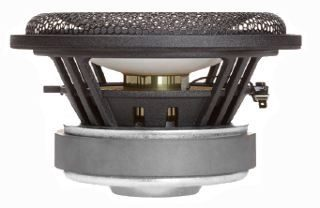open a bigger photo

recommended cabinet 1:
closed cabinet with 4,3 L volume
from 82/52 Hz (-3dB/-8dB)

recommended cabinet 2:
12,3 L volume bass reflex cabinet
with HP50 reflex tube, 22 cm long.
from 46/35 Hz (-3dB/-8dB).

## Accuton Thiel C173-11-095

order no. not-available- not available
16 cm bass-midrange driver with ceramic cone.
• power handling (continuous/programme) = 120 W
• frequency range = 35-2500 Hz
• resonance frequency fs = 30 Hz
• impedance R = 12 Ohm
• sound pressure level SPL = 87 dB (2,83V; 1m)
• DC resistance Re = 11 Ohm
• force factor BL = 10,2 N/A
• voice coil inductance L = 1 mH
• effective piston radiating area Sd = 130 cm2
• effective mechanical mass incl. air load mms = 16 g
• equivalent volume of compliance Vas = 34 l
• total Q factor Qts = 0,3 (Qms=4,23, Qes=0,32)
• voice coil diameter = 38 mm
• maximum peak linear excursion vibration xlin = +/- 5 mm
• mounting diameter d = 145,6 mm
• overall diameter d = 173 mm
• mounting depth (not countersunk) t = 94,5 mm
• mass m = 2,28 kg
PDF data sheetopen a bigger photo

recommended cabinet 1:
closed cabinet with 21 L volume
from 57/36 Hz (-3dB/-8dB)

recommended cabinet 2:
51 L volume bass reflex cabinet
with HP70 reflex tube, 20 cm long.
from 34/26 Hz (-3dB/-8dB).

## Accuton Thiel S280-18-282n

order no. not-available- not available
27 cm bass driver with sandwich cone.
• power handling (continuous/programme) = 150 W
• frequency range = 18-500 Hz
• resonance frequency fs = 18 Hz
• impedance R = 20 Ohm
• sound pressure level SPL = 88 dB (2,83V; 1m)
• DC resistance Re = 18,5 Ohm
• force factor BL = 25,4 N/A
• voice coil inductance L = 1,96 mH
• effective piston radiating area Sd = 408 cm2
• effective mechanical mass incl. air load mms = 83 g
• equivalent volume of compliance Vas = 226 l
• total Q factor Qts = 0,26 (Qms=6,87, Qes=0,27)
• voice coil diameter = 55 mm
• maximum peak linear excursion vibration xlin = +/- 6 mm
• mounting diameter d = 251,2 mm
• overall diameter d = 280 mm
• mounting depth (not countersunk) t = 132 mm
• mass m = 3,8 kg
PDF data sheet

 Tweeter Mids Woofer Subwoofer Passive Radiators no longer sold
The cabinet volume calculations are executed considering an external resistance of 0.3 Ohm. We are using, if no other data is known or available, the information supplied by the manufacturer.

Please note: the frequency response doesn't say much about the cut-off frequency inside the cabinet! Therefore, we mostly give you 2 examples with dimensioning considerations and an indication, how low the loudspeaker will reproduce.

Home